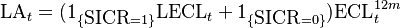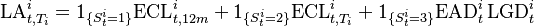# Loss Allowance

## Definition

Loss Allowance, in the context of IFRS 9 , is an estimate linked to expected credit losses on a financial asset that is applied to reduce the carrying amount of the financial asset in the Statement of Financial Position.

The required Loss Allowance equals the 12-month Expected Credit Losses on the financial asset if there has been no Significant Increase in Credit Risk since purchase or origination. The Loss Allowance increases to the Lifetime Expected Credit Losses if such an increase has occurred at the reporting date.

Collectively Allowances denotes the stock of lending exposure loan loss provisions that has been recognised in the Balance Sheet of the credit institution, in accordance with the applicable accounting framework

## Formula$\mbox{LA}_t = (1_{ \{ \mbox{SICR} = 1 \} } \mbox{LECL}_{t} + 1_{ \{ \mbox{SICR} = 0 \} } ) \mbox{ECL}^{12m}_{t}$

where the indicator SICR selects the Stage 1 Assets or Stage 2 Assets and Stage 3 Assets categories.

In terms of Stages, the same formula can be decomposed as$\mbox{LA}_{t,T_i}^{i} = 1_{ \{ S_t^{i} = 1 \} } \mbox{ECL}^{i}_{t,12m} + 1_{ \{ S^{i}_t = 2 \} } \mbox{ECL}_{t,T_i}^{i} + 1_{ \{ S^{i}_t = 3 \} } \mbox{EAD}_{t}^{i} \, \mbox{LGD}_{t}^{i}$